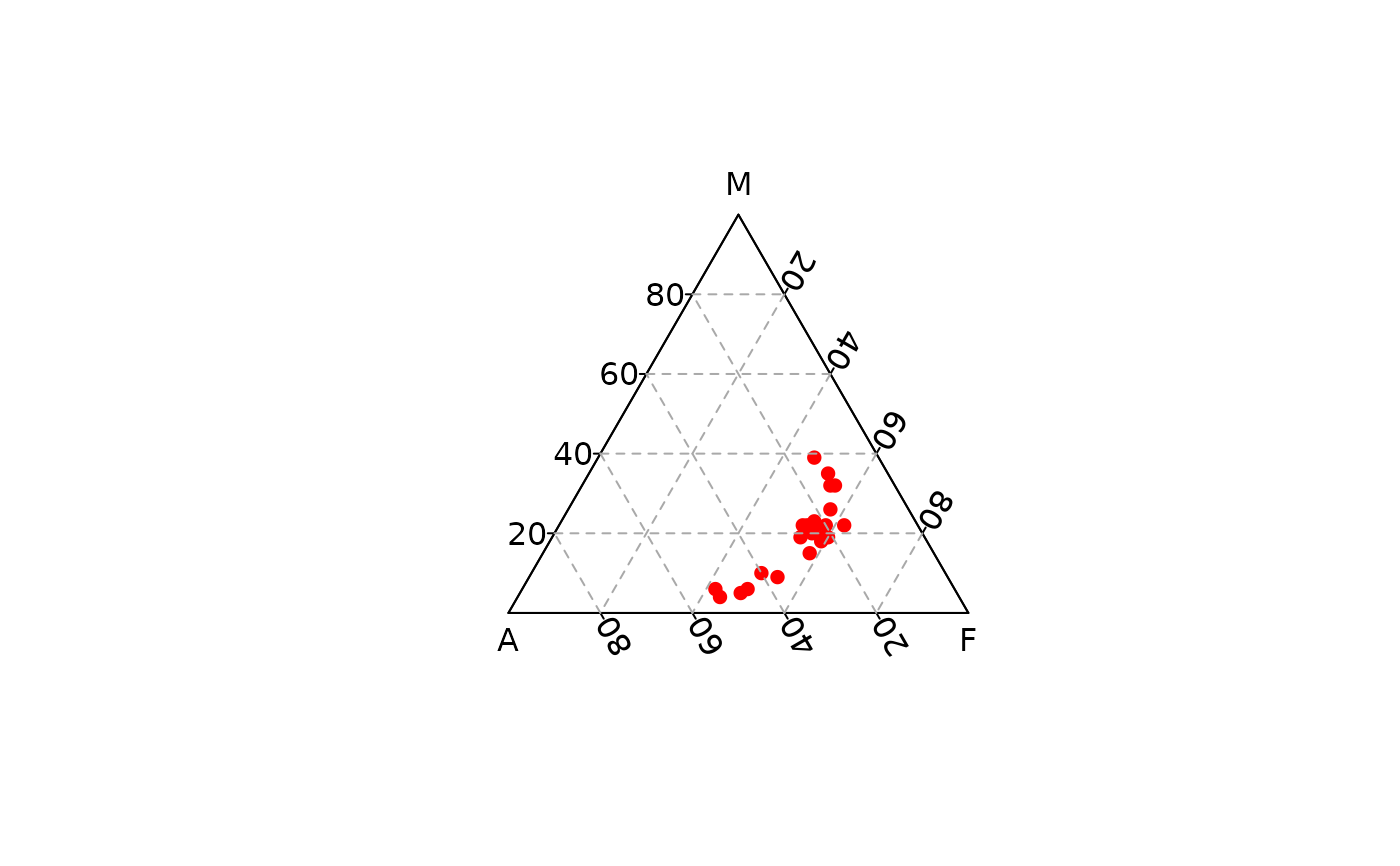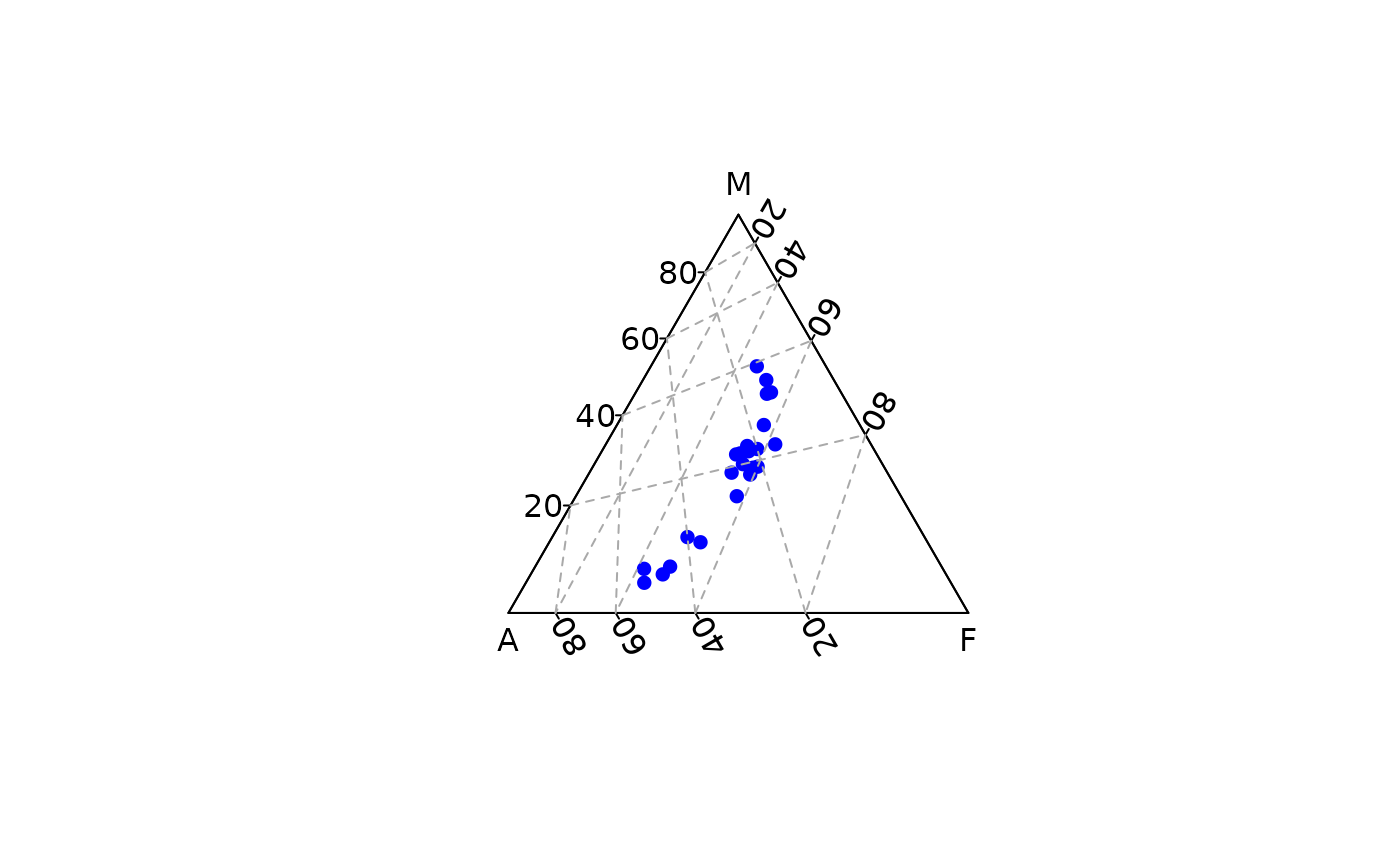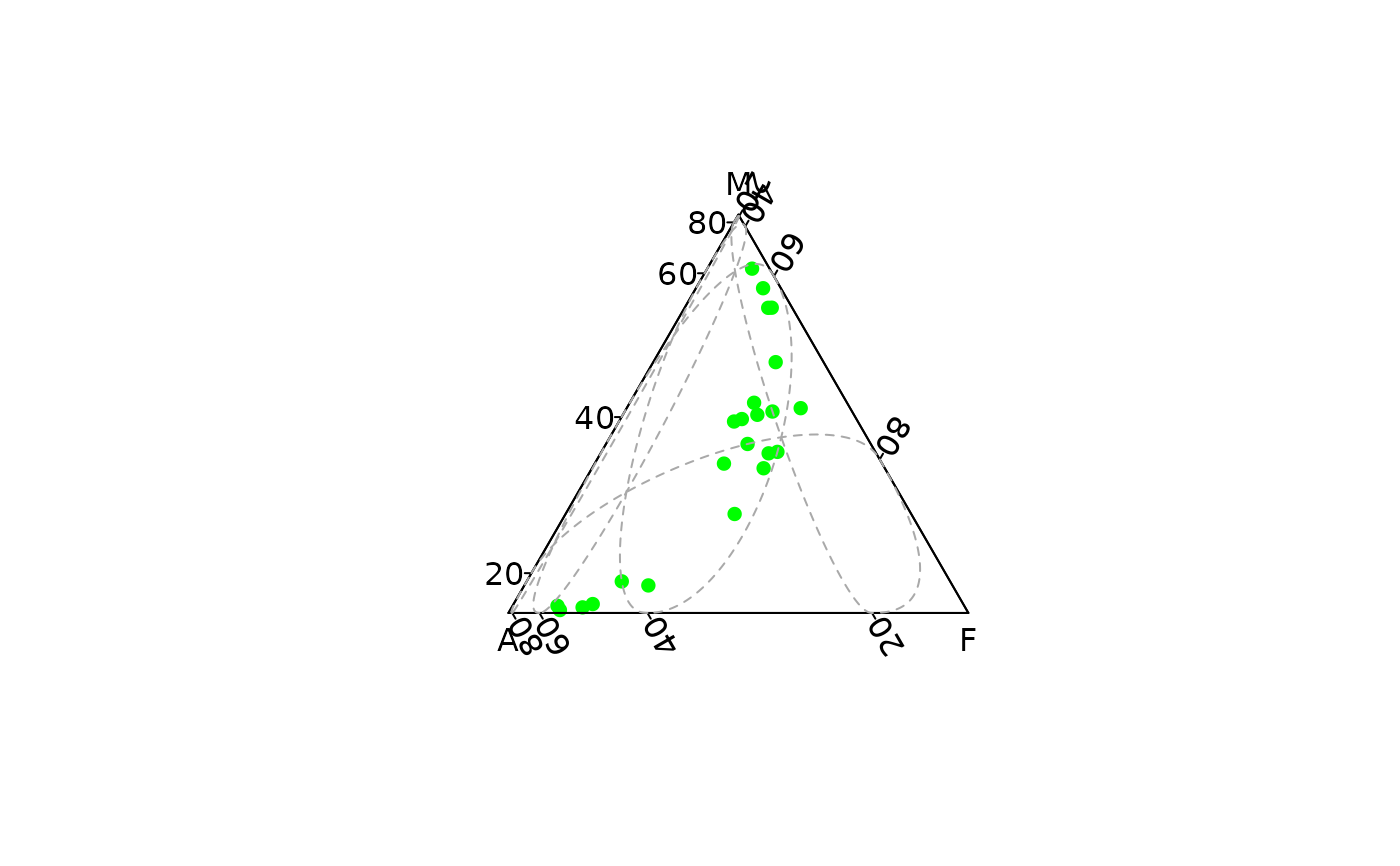Adds a triangular grid to an existing plot.

## Usage

ternary_grid(
primary = NULL,
secondary = NULL,
col.primary = "darkgray",
col.secondary = "lightgray",
lty.primary = "dashed",
lty.secondary = "dotted",
lwd.primary = 1,
lwd.secondary = lwd.primary
)

## Arguments

primary

An integer specifying the number of cells of the primary grid in x, y and z direction.

secondary

An integer specifying the number of cells of the secondary grid in x, y and z direction.

col.primary, col.secondary

A character string specifying the color of the grid lines.

lty.primary, lty.secondary

A character string or numeric value specifying the line type of the grid lines.

lwd.primary, lwd.secondary

A non-negative numeric value specifying the line width of the grid lines.

## Value

ternary_grid() is called it for its side-effects.

Other graphical elements: ternary_axis(), ternary_box(), ternary_pairs(), ternary_plot(), ternary_title()

N. Frerebeau

## Examples

## Blank plot
ternary_plot(NULL)## Compositional data
coda <- data.frame(
X = c(20, 60, 20, 1/3),
Y = c(20, 20, 60, 1/3),
Z = c(60, 20, 20, 1/3)
)

## Ternary plot
ternary_plot(coda, pch = 16, col = "red")ternary_plot(coda, panel.first = ternary_grid(5, 10))## Zoom
ternary_plot(coda, xlim = c(0.5, 1), panel.first = ternary_grid())ternary_plot(coda, ylim = c(0.5, 1), panel.first = ternary_grid())ternary_plot(coda, zlim = c(0.5, 1), panel.first = ternary_grid())## Color according to a supplementary variable
## Data from Aitchison 1986
col <- grDevices::colorRampPalette(c("red", "blue"))(nrow(arctic))
ternary_plot(arctic, panel.first = ternary_grid(), pch = 16, col = col)